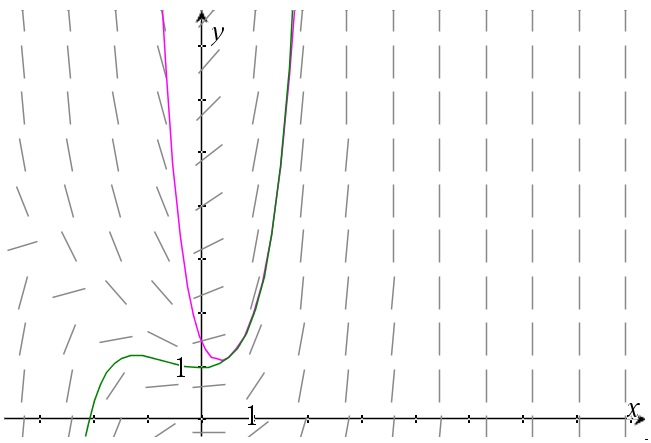# Using Taylor series to solve differential equations

Up a level : Differential Equations
Previous page : First order linear differential equations - a couple of examples
Next page : Simple harmonic motion again – using Taylor seriesI bit further away along the pages we solve the equation$y' - xy = {x^2}$

You can have a look at it here. The problem we quickly encountered was that the solution is a non-elementary function. Let us tackle this in another way. Previously, in the section on power series we were able to solve the equation y´=y by assuming the solution could be expressed as a power series. Let us attempt something similar here.

Say we want a solution that goes through the point (1, 2). So we start with assuming we have a solution that can be expressed with a Taylor series, i.e. that$\begin{gathered} y = f(x) = \sum\limits_{k = 0}^\infty {\frac{{{f^{(k)}}(a)}}{{k!}}{{(x - a)}^k}} \hfill \\ \quad = f(a) + f'(a)(x - a) + \frac{{f''(a)}}{{2!}}{(x - a)^2} + \frac{{f'''(a)}}{{3!}}{(x - a)^3} + ... \hfill \\ \end{gathered}$

In this case we have that$y(1) = f(a) = f(1) = 2$

and that$y' = {x^2} + xy$

so$y'(1) = {1^2} + 1 \cdot 2 = 3 = f'(a)$

Differentiating our differential equation once gives us$y'' = 2x + y + xy'$

and thus$y''(1) = 2 \cdot 1 + 2 + 1 \cdot 3 = 7$

Differentiate again$y''' = 2 + y' + y' + y'' = 2 + 2y' + y''$

so$y'''(1) = 2 + 2 \cdot 3 + 7 = 15$

Differentiate again$\begin{gathered} {y^{(4)}} = 3y'' + y''', \hfill \\ {y^{(4)}}(1) = 3 \cdot 7 + 15 = 36 \hfill \\ \end{gathered}$

So, an approximate solution should be$y = 2 + 3(x - 1) + \frac{7}{2}{(x - 1)^2} + \frac{{15}}{6}{(x - 1)^3} + \frac{{36}}{{24}}{(x - 1)^4}$

In the graph below we can see the slope field, the particular solution in green (found in the above mentioned page) and the approximate solution in violet.If we try that for x=4 we get y=12.5, that is rather far from the about 13.96 we should get according to the exact solution. If we add some more terms we get$\begin{gathered} {y^{(5)}} = 4y'' + xy''', \hfill \\ {y^{(5)}}(1) = 3 \cdot 15 + 1 \cdot 36 = 96 \hfill \\ \end{gathered}$

From this point on we have that$\begin{gathered} {y^{(n)}} = (n - 1){y^{(n - 1)}} + x{y^n}, \hfill \\ {y^{(n)}}(1) = (n - 1){y^{(n - 1)}}(1) + {y^n}(1) \hfill \\ \end{gathered}$

This gives us the sequence 2, 3, 7, 15, 36, 96, 267, 852 and so on. We can use these values to get a better approximation.  Using our exact method we get 13.96225, using 15 terms in our series expansion we get 13.69221, so quite close.Up a level : Differential Equations
Previous page : First order linear differential equations - a couple of examples
Next page : Simple harmonic motion again – using Taylor seriesLast modified: Apr 1, 2019 @ 16:31